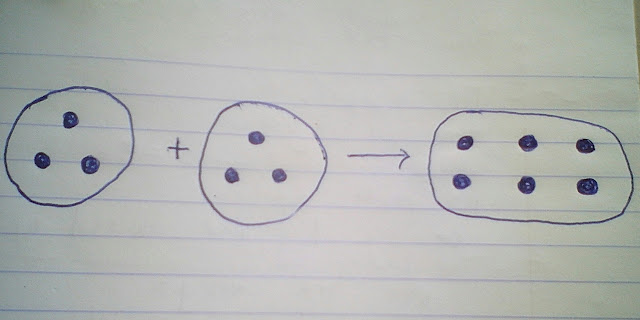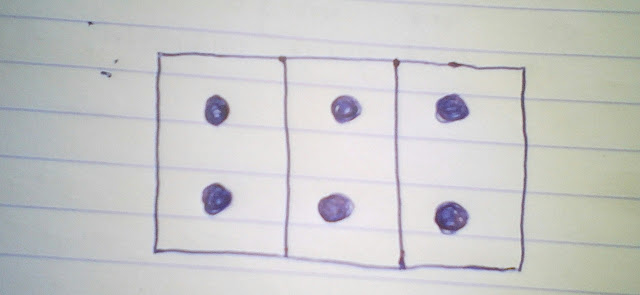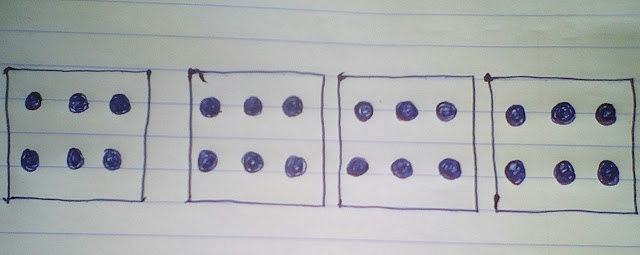# How To Teach A Child Multiplication Of Whole Numbers

TEACHING AND LEARNING MATERIALS
You can use countable objects such as seeds, marbles, beads, bottle tops etc.

But in this lesson, I will use marbles.

ACTIVITY 1
Explain the concept of multiplication as repeated addition.
Example, 2×3 means 2 groups of 3 objects.That is, 3+3=6
Which means 2×3=6

ACTIVITY 2
Guide the child to perform practical activities using countable objects like the marbles to show that the product of three (3) numbers does not change when the factors are regrouped
( 1- digit factors only)

Example; 4×(3×2)=(4×3)×2

i) Make an array of 3 groups of 2 objects giving altogether 6 objects.ii) Make an array of 4 groups of 6 objectsCount to find the result that is 24.

ACTIVITY 3
Regroup the factors for the child to find the results by following the same procedure.
That is (4×3)×2.

CONCLUSION
Go over the main points with the child and give him/her the questions below to solve.

Question
Complete the following sentences by regrouping the factors
i) (1×2)×3=
ii) (3×4)×5=
iii) (4×5)×6=
iv) (5×6)×7=